# In Situ Measurement of Vacuum Window Birefringence using 25Mg+ Fluorescence

W. H. Yuan1, H. L. Liu1, W. Z. Wei1, Z. Y. Ma1, P. Hao1, Z. Deng1, K. Deng1, J. Zhang1, Z. H. Lu1
Video Coming Soon

Yuan, W. H., Liu, H. L., Wei, W. Z., Ma, Z. Y., Hao, P., Deng, Z., Deng, K., Zhang, J., Lu, Z. H. In Situ Measurement of Vacuum Window Birefringence using 25Mg+ Fluorescence. J. Vis. Exp. (160), e61175, doi:10.3791/61175 (2020).

### Abstract

Accurate control of the polarization states of laser light is important in precision measurement experiments. In experiments involving the use of a vacuum environment, the stress-induced birefringence effect of the vacuum windows will affect the polarization states of laser light inside the vacuum system, and it is very difficult to measure and optimize the polarization states of the laser light in situ. The purpose of this protocol is to demonstrate how to optimize the polarization states of the laser light based on the fluorescence of ions in the vacuum system, and how to calculate the birefringence of vacuum windows based on azimuthal angles of external wave plates with Mueller matrix. The fluorescence of 25Mg+ ions induced by laser light that is resonant with the transition of |32P3/2,F = 4, mF = 4→ |32S1/2,F = 3, mF = 3is sensitive to the polarization state of the laser light, and maximum fluorescence will be observed with pure circularly polarized light. A combination of half-wave plate (HWP) and quarter-wave plate (QWP) can achieve arbitrary phase retardation and is used for compensating the birefringence of the vacuum window. In this experiment, the polarization state of the laser light is optimized based on the fluorescence of 25Mg+ ion with a pair of HWP and QWP outside the vacuum chamber. By adjusting the azimuthal angles of the HWP and QWP to obtain maximum ion fluorescence, one can obtain a pure circularly polarized light inside the vacuum chamber. With the information on the azimuthal angles of the external HWP and QWP, the birefringence of the vacuum window can be determined.

### Introduction

In many research fields such as cold atom experiments1, measurement of the electric dipole moment2, test of parity-nonconservation3, measurement of vacuum birefringence4, optical clocks5, quantum optics experiments6, and liquid crystal study7, it is important to precisely measure and accurately control the polarization states of laser light.

In experiments involving the use of a vacuum environment, the stress-induced birefringence effect of vacuum windows will affect the polarization states of laser light. It is not feasible to put a polarization analyzer inside the vacuum chamber to directly measure the polarization states of the laser light. One solution is to use atoms or ions directly as an in situ polarization analyzer to analyze the birefringence of vacuum windows. The vector light shifts of Cs atoms8 are sensitive to the degrees of linear polarization of the incidence laser light9. But this method is time consuming and can only be applied to the linearly polarized laser light detection.

Presented is a new, quick, precise, in situ method to determine the polarization states of laser light inside the vacuum chamber based on maximizing single 25Mg+ fluorescence in an ion trap. The method is based on the relationship of the ion fluorescence to the polarization states of the laser light, which is affected by the birefringence of the vacuum window. The proposed method is used for detecting the birefringence of vacuum windows and degrees of circular polarization of laser light inside a vacuum chamber10.

The method is applicable to any atoms or ions whose fluorescence rate is sensitive to the polarization states of laser light. In addition, while the demonstration is used to prepare a pure circularly polarized light, with the knowledge of the birefringence of the vacuum window, arbitrary polarization states of laser light can be prepared inside the vacuum chamber. Therefore, the method is quite useful for a wide range of experiments.

### Protocol

1. Set up the reference directions for polarizers A and B

1. Put polarizer A and polarizer B into the laser beam (280 nm fourth harmonic laser) path.
2. Ensure that the laser beam is perpendicular to the surfaces of the polarizers by carefully adjusting the polarizer holders to keep the back-reflection light coincident with the incident light.
NOTE: All the following alignment procedures for the optics components must follow the same rule. The placement of polarizer A and B in the laser path is not important. The spacing between them should be large enough for the future convenient adjustment.
3. Put a power meter behind polarizer A and rotate the polarizer to maximize the output power. Define the azimuthal angle (see Results and Discussion) of the optical axis of polarizer A as 0°. Define the clockwise direction as the positive direction and the counterclockwise direction as the negative direction when observing along the direction of light propagation.
1. Use a stepper motor rotation stage to hold polarizer A and put the power meter behind polarizer A to record the rotation angles and the output laser powers. Fit the angle vs power curve with a sinusoidal function; the maximum output power position of polarizer A is 0° azimuthal angle position.
4. Put the power meter behind polarizer B and rotate polarizer B to maximize the output power. The azimuthal angle of the optical axis of polarizer B is then also 0°.
1. Use another stepper motor rotation stage to hold polarizer B and put the power meter behind polarizer B to record the rotation angles and the output laser powers. Fit the angle vs power curve with a sinusoidal function; the maximum output power position of polarizer B is 0° azimuthal angle position (see Figure 1).

2. Set up the reference directions for the azimuthal angles of the waveplates

1. Put an HWP into the beam path between polarizer A and polarizer B and rotate the HWP to maximize the output power. The azimuthal angle of the optical axis of the HWP is then 0°.
1. Use a stepper motor rotation stage to hold the HWP and put the power meter behind polarizer B to record the rotation angles and the output laser powers. Fit the angle vs power curve with a sinusoidal function; the maximum output power position of the HWP is 0° azimuthal angle.
2. Put a QWP into the beam path between the HWP and polarizer B, rotate the QWP to maximize the output power. The azimuthal angle of the optical axis of the QWP is then 0°.
1. Use a stepper motor rotation stage to hold the QWP and put the power meter behind polarizer B to record the rotation angles and the output laser powers. Fit the angle vs power curve with a sinusoidal function; the maximum output power position of the QWP is 0° azimuthal angle position.
3. Remove polarizer B and the power meter from the beam path. Use two mirrors to direct laser beam into the vacuum chamber that houses an ion trap to interact with 25Mg+ ions.
NOTE: The laser propagation direction should be along the magnetic field direction inside the vacuum chamber. A magnetic field is used to define the quantization axis of the ions.

3. Doppler cooling of single 25Mg+ ions

1. Turn on the 532 nm ablation laser, which is a Q-switched Nd:YAG laser. Its repetition rate is 1 kHz, with pulse energy of 150 µJ. The ablation laser irradiates a magnesium wire target surface inside the vacuum chamber, and then magnesium (Mg) atoms are ejected from the target surface.
NOTE: The power supply for the ion trap should be turned on.
2. At the same time, turn on the 285 nm ionization laser to ionized Mg atoms. The ionization laser is a fourth harmonic laser with an output power of 1 mW. The ionization laser will illuminate the center of the ion trap.
3. Make sure only one ion is trapped in the ion trap by looking at the image of an electron multiplied charged coupled device (EMCCD). An example image showing trapped ions is shown in Figure 2. Each bright spot is one ion. If there is more than one ion in the trap, turn off the power supply of the ion trap to release the ions. Then repeat steps 3.1-3.2 until only one (i.e., single) ion is trapped.
NOTE: The homemade imaging system of the EMCCD consists of four lenses, and its magnification is 10x. The ion spacing is about 2-10 µm and the pixel spacing of the EMCCD is 16 µm. The EMCCD can, therefore, be used to identify the existence of one single ion.
4. Set the magnetic field to be 6.5 Gauss by adjusting the current of Helmholtz coils. The magnetic field is measured by comparing the different frequencies between the two ground state transitions,and. For details of the method please refer11.

4. Lock the 280 nm Doppler cooling laser frequency to a wavelength meter12

1. Scan the frequency of the 280 nm laser and count the fluorescence photon numbers collected by a photon multiplier tube (PMT) by a frequency counter. At the same time, record the frequency of the laser using a wavelength meter. Find the resonant frequency ν0 where the fluorescence rate reaches a maximum.
NOTE: The fluorescence counts will increase when the laser frequency is moving close to the ion resonant frequency and will reach a maximum at the resonant frequency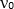.
2. Lock the laser frequency to the wavelength meter using a digital servo control program that is running on an accompanying computer. Click on the Lock button on the program graphic interface when the wavelength meter shows a reading of.

5. Set the intensity of the laser to equal the saturation intensity12

1. Change the power of the laser by adjusting the driving power of an acousto-optic-modulator (AOM), which is used in the beam path to change the frequency and power of the laser. Record the power and the fluorescence counts.
2. Fit the curve of the power and the fluorescence counts with Equation (6), and obtain the saturation power.
3. Set the laser power toby adjusting the driving power of the AOM.

6. Measure the birefringence of the vacuum window.

1. Alternately, adjust the azimuthal angles of the HWP and the QWP to maximize the fluorescence counts. Record the azimuthal angles of the HWP and the QWP at maximum counts, which are α and β.
1. Use the stepper motor rotation stages to rotate the HWP and the QWP and record the rotation angles and the corresponding fluorescence counts.
2. Use Equation (4) and Equation (5) to calculate the birefringence of the vacuum window θ and.

### Representative Results

Figure 3 shows the beam path of the experiment. Polarizer B in Figure 3a is removed after angle initialization (Figure 3b). The laser passed through a polarizer, an HWP, a QWP, and the vacuum window, sequentially. The Stokes vector of laser is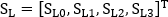, whereis the normalized laser power. The Stokes vector should be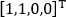after passing the polarizer, which means the laser was linearly polarized. The Mueller matrices for the polarizer, HWP, QWP, and vacuum window wereand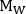, respectively. Finally the ion was excited by the laser and the fluorescence was collected by a PMT. The Stokes vector of the laser inside the vacuum chamber waswhere R is the rotational matrix, α and β are the azimuthal angles of the HWP and the QWP, respectively. The Mueller matrix of each optical components and the rotational matrix are given below:From Equation (1), the Stokes vector of the laser inside the vacuum chamber is:Here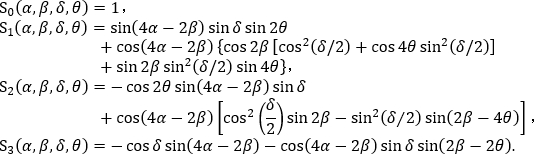Specifically, when the laser is circularly polarized, that is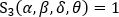, there must be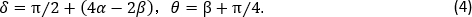or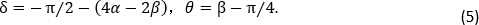The two results correspond to whether we define the fast axis angle as 0° or the slow axis angle as 0°. They were equivalent when the fast axis was exchanged with the slow axis. Equation (4) and Equation (5) are the relationships between the azimuthal angles of the waveplates and the birefringence of the vacuum window when the laser in the vacuum chamber is circularly polarized.

To determine the polarization states of the light inside the vacuum chamber, one should know the relationship between the polarization states of the light and the fluorescence counts. Because 25Mg+ ion has 48 Zeeman levels, as shown in Figure 4, analytical solutions cannot be derived from the rate equations. But these can be simulated by numerical program, and the numerical results are shown in Figure 5. In the figure, the relationships between the polarization states and the fluorescence counts under different light intensities are shown. From the relationships, we know the polarization state of the light inside the vacuum chamber iswhen the fluorescence counts are maximized. At this position, the fluctuation of the fluorescence count is <2%.

In the protocol section 5, the intensity of the laser is set to the saturation intensity. When the frequency of the laser is fixed, the fluorescence count depends on the intensity of the laser. The relation is14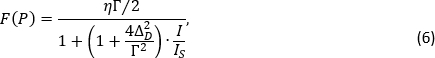where ΔD is the laser detuning from the resonant frequency,is the natural linewidth of the magnesium ion's upper energy level.and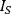are the laser intensity and the saturation intensity, respectively. The intensity and power have relationship of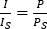, so the light intensity isif the power is. Figure 6 shows the relationship of the laser power and the fluorescence counts under different detuning frequencies. We can fit the curves with Equation (6) to obtain the saturated power.

By fixing the azimuthal angle of one waveplate and rotating the other, and recording the angles and the fluorescence counts, we got Figure 7. The red line is the theoretical result and the black dots with error bars are the experimental results. They agree with each other very well, demonstrating the reliability of the method.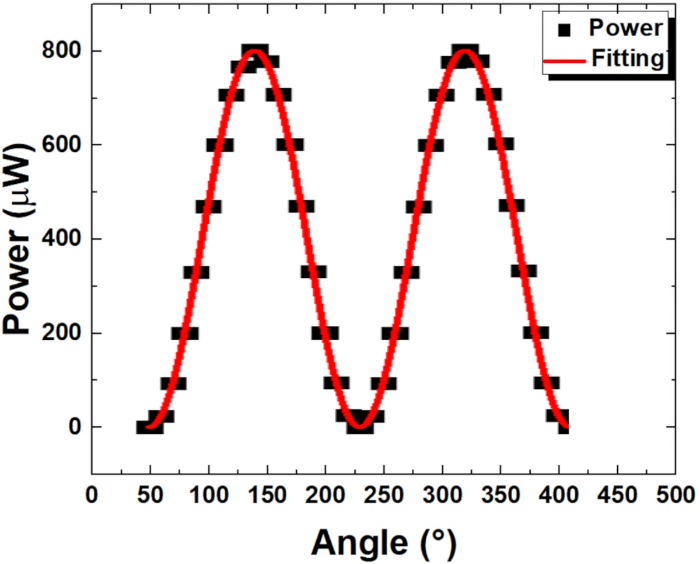Figure 1: Relationship between the azimuthal angle of polarizer B and the laser power. Rotate the azimuthal angle of polarizer B and record the laser power. The fitted cure is a sinusoidal function. The azimuthal angle of polarizer B is 0° when the power is maximum. There are two maximum points corresponding to two polarization axis positions with angle difference of 180°. Please click here to view a larger version of this figure.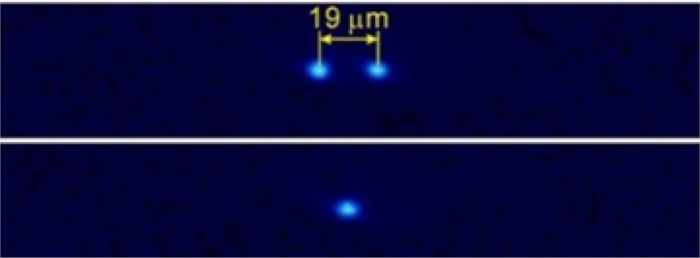Figure 2: Picture of trapped ions taken by the EMCCD. The first row shows the example of two trapped ions, and the second row shows an example of one trapped ion. Each bright spot corresponds to one ion. Please click here to view a larger version of this figure.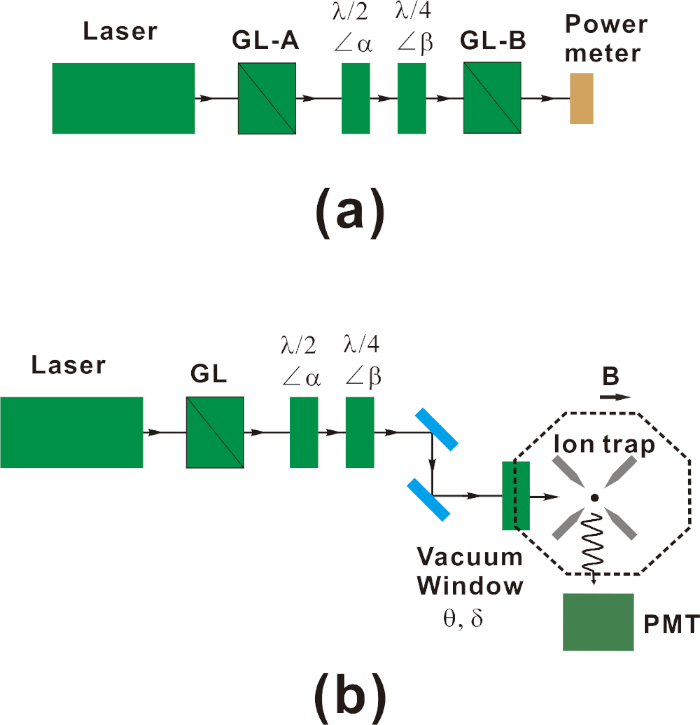Figure 3: Schematics for the experimental set up. (a) The experimental setup for defining the azimuthal angles of different optical components. Polarizer A (GL-A) was used to initialize angles of each components, and polarizer B (GL-B) was used to analyze this initialization./2 is HWP,/4 is QWP. (b) The experimental setup for determining the birefringence of the vacuum window. A 280 nm laser passes through a polarizer A (GL-A), an HWP, a QWP and vacuum window, and then illuminates 25Mg+ ions. Please click here to view a larger version of this figure.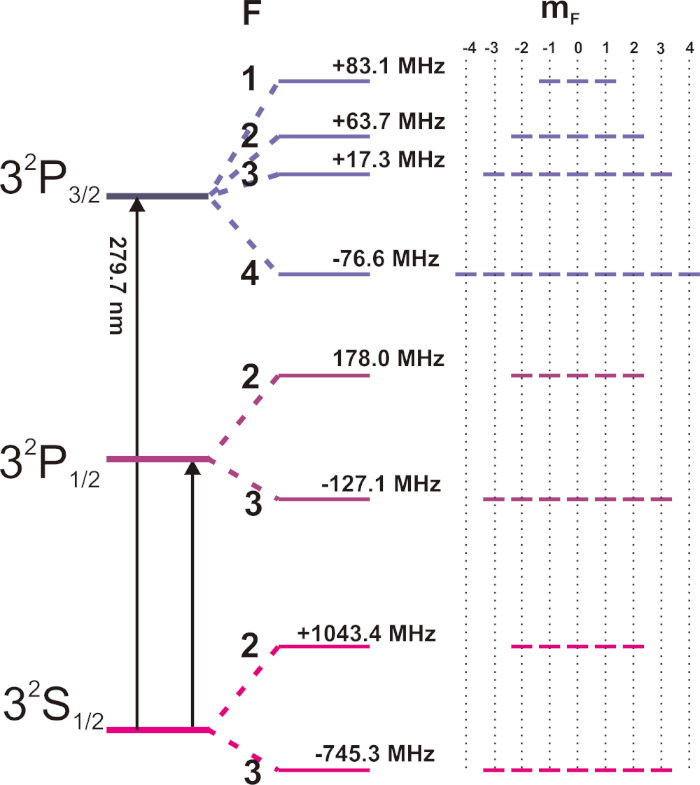Figure 4: The relevant energy levels of 25Mg+ ion. F is the total angular momentum quantum number, and mF is the magnetic quantum number. Different mF values correspond to different Zeeman levels that have different energy values under a magnetic field. There are 48 Zeeman levels in the figure (each is shown with a short horizontal lines) that are used for simulating the population distribution. Please click here to view a larger version of this figure.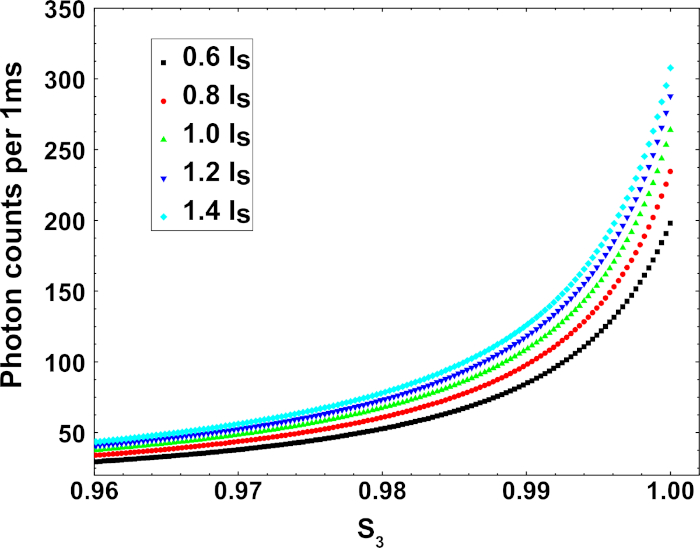Figure 5: Simulation results showing the relationship of the laser polarization state and the fluorescence counts with different laser intensities. The magnetic field was fixed at 6.5 G, which is consistent with our experimental parameter. This figure was modified from Yuan et al.10. Please click here to view a larger version of this figure.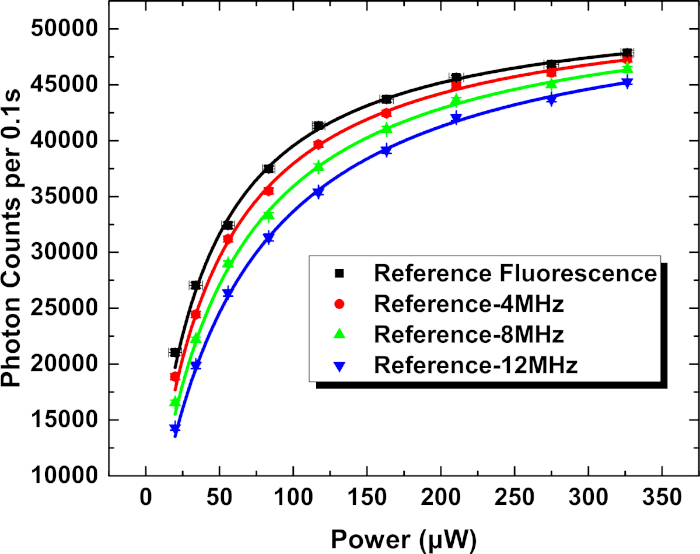Figure 6: Fluorescence count per 0.1 s vs laser power for different laser frequency detuning ΔD. This figure was modified from Yuan et al.13. Please click here to view a larger version of this figure.Figure 7: The relationship of the fluorescence counts with the azimuthal angles of the wave plates. (a) Varying the azimuthal angles of the HWP with the angle of the QWP set at 149°. (b) Varying the azimuthal angles of the QWP with the angle of the HWP set at 2.6°. The black dots are the experimental results, the error bars were determined by the standard deviations of the fluorescence count fluctuations. The red lines are the theoretical calculation results based on simulation results. This figure was modified from Yuan et al.10. Please click here to view a larger version of this figure.

### Discussion

This manuscript describes a method to perform in situ measurement of the birefringence of the vacuum window and the polarization states of the laser light inside the vacuum chamber. By adjusting the azimuthal angles of the HWP and the QWP (α and β), the effect of the birefringence of the vacuum window (δ and θ) can be compensated so that the laser inside the vacuum chamber is a pure circularly polarized light. At this point, there exists a definite relationship between the birefringence of the vacuum window and the azimuthal angles of the HWP and the QWP, from which we can infer the birefringence of the vacuum window. Measurement errors of the azimuthal angles affect the accuracy of the birefringence measurement. Therefore, in the initialization of the waveplate azimuthal angle step, the stepper motor rotation stage should be sufficiently accurate (~0.001°). As an alternative, other common phase retarder, such as crystal wave plates, liquid-crystal-based wave plates or electro-optic modulators, could be used for compensating the birefringence of the vacuum window. Some other systematic uncertainties will also affect the measurement accuracy, such as the frequency and the power stability of laser, dark count of PMT, shot noise, and so on. These are discussed in Yuan et al.10.

To perform the method accurately, one needs to prepare lasers to ionize Mg atoms and irradiate the 25Mg+, a pair of HWP and QWP for adjusting the polarization states of the laser, two Glan-Taylor polarizers to guarantee and test polarization states, ion trap for ion storage, mirrors, Mg target material, PMT for counting the photon, EMCCD for imaging the ion in the trap, stepper motor rotation stages to adjust the azimuthal angles of polarizers and waveplates.

In vacuum-based experiments, such as optical clocks5, cold atoms1, atom interferometers15, quantum optics experiments6, this method can be used to in situ measure the birefringence of the vacuum window. The birefringence is caused by the stress on the vacuum window; hence it will be different when the temperature changes. As the method is much simpler and faster, it can be applied to compensate the thermal effect in real-time by feedback to the waveplates.

The success of this method hinge on the extremely high sensitivity of the fluorescence rate to the laser polarization states. There might have atom or ion systems whose fluorescence rates are not sensitive to the laser polarization states. Therefore, in other atom or ion systems, for the method to work, simulation of the relationship of the laser polarization states and the fluorescence counts needs to be performed to determine whether this method is suitable. Simulation is based on rate equations. More steps and smaller step size will make the result more accurate, with the downside of longer measurement time. The steps should be small enough, in our experience it is about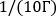. The population of each level will reach stable state after sufficient time. The proper time is associated with the energy level structures of specific ion or atom. As for 25Mg+ ion, the simulation contains 48 energy levels, so 106 times steps are suitable. For other atoms or ions, the population should be first simulated to determine the suitable step number.

### Disclosures

The authors have nothing to disclose.

### Acknowledgments

This work was partially supported by the National Key R&D Program of China (Grant No. 2017YFA0304401) and the National Natural Science Foundation of China (Grant Nos. 11774108, 91336213, and 61875065).

### Materials

 Name Company Catalog Number Comments 280 nm Doppler cooling laser Toptica SYST DL-FHG Pro 280 Doppler cooling laser 285 nm ionization laser Toptica SYST DL-FHG Pro 285 ionization laser Ablation laser Changchun New Industries Optoelectronics Technology EL-532-1.5W Q-switched Nd:YAG laser AOM Gooch & Housego AOMO 3200-1220 wavelengh down to 257 nm EMCCD camera Andor iXon3 897 imaging of 25Mg+ in ion trap Glan-Taylor polarizer Union Optic Custom distinction ratio 1e-6 Half waveplate Union Optic Custom made of quartz Photon multiplier tube Hamamatsu H8259-09 fluorescent counting Power meter Thorlabs PM100D laser power monitor Quarter waveplate Union Optic Custom made of quartz Mirror Union Optic Custom dielectric coated for 280 nm Stepper motor roation stage Thorlabs K10CR1/M rotating wave plates Vacuum chamber Kimball Physics MCF800-SphSq-G2E4C4 made of Titanium Vacuum window Union Optic Custom made of fused silica